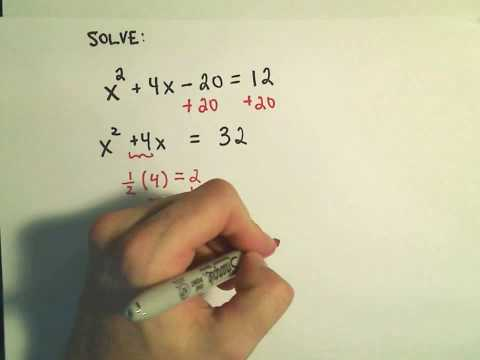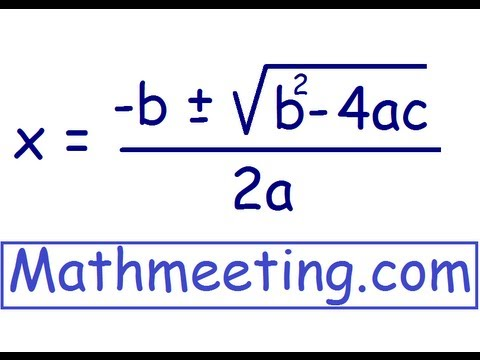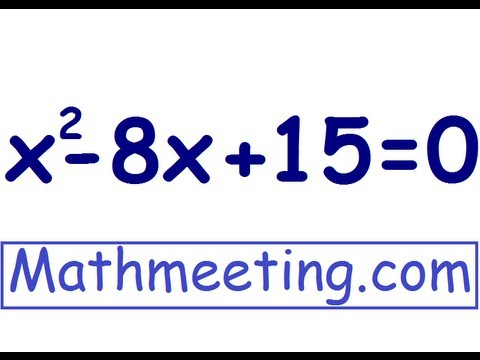## Quadratic equation

Subject: Compulsory Maths

Find Your Query

#### Overview

An equation like ax2+ bx + c = 0 where a≠ 0, which contains only one variable and '2' as its highest power is called a quadratic equation. There are two values of the variable in any quadratic equation.

##### Quadratic equation

An equation like ax2+ bx + c = 0 where a ≠ 0, which contains only one variable and '2' as its highest power is called a quadratic equation. There are two values of the variable in any quadratic equation. The roots of the equations are :

$$x = \frac {-b \pm \sqrt {b^2 -4ac}}{2a}$$#### Quadratic equation are of two types

i. Pure quadratic equation:

A quadratic equation of the form ax2+ c = 0, where the middle term containing power 1 is missed is known as a pure quadratic equation.

e.g. x2 = 9 or, x2 - 9 = 0
i.e. ax2 + c = 0 ( a ≠ o, c = 0 )

ii. Adfected quadratic equation:

The standard form of quardic equation is known as adfected quadratic equation.

ax2 + bx + c = 0 is adfected quadratic equation.

e.g. x2 - 9x - 15 = 0
i.e. ax2 + bx + c = 0 ( a ≠ o, b ≠ o)

#### Solution of quadratic equation

Quadratic equation is a second-degree equation of one variable. So we get two solutions of the variable contained by this equation. The solution of a quadratic equation is known as the roots. Hence, a quadratic equation has two roots. The solution of roots which are obtained from the quadratic equation should satisfy the equation. There are three major methods for solving quadratic equation, which are

1. Factorization method
2. Completing square method
3. Using formula

a) Solving a quadratic equation by factorization method:

In this method, the quadratic equation ax2 + bx+ c = 0 is factorized and expressed as the product of two linear factors. Again, the two linear factors are also solved to get the solution of the equation. the roots that is obtained from the equation should satisfy the given quadratic equation.

b) Solving a quadratic equation by completing square method:

We transpose the constant term (c) to the R.H.S and the remaining parts ax2 + bx is expressed in the perfect square expression.

c) Solving a quadratic equation by using formula:

In this method, we have obtained two roots as $\frac{-b+\sqrt{b^2-4ac}}{2a}$ and $\frac{-b-\sqrt{b^2-4ac}}{2a}$

the two roots of the quadratic equation ax2+ bx+ c = 0 can be obtained using a direct formula,

x = $\frac{-b±\sqrt{b^2-4ac}}{2a}$

'a' is the coefficient of x2

'b' is the coefficient of x and

'c' is the constant term.

While solving any quadratic equation using the formula, first we simplify and bring the equation in the simplest form. Then we compare with ax2+ bx+ c = 0 and find a, b and c. Finally, we use x = $\frac{-b±\sqrt{b^2-4ac}}{2a}$ and find two roots of the given quadratic equation.

##### Things to remember
• There are two types of quadratic equation. They are pure and adfected quadratic equation.
•  There are three major methods for solving quadratic equation, which are

1. Factorization method
2. Completing square method
3. Using formula
• It includes every relationship which established among the people.
• There can be more than one community in a society. Community smaller than society.
• It is a network of social relationships which cannot see or touched.
• common interests and common objectives are not necessary for society.
##### Videos for Quadratic equation##### Completing the Square to Solve Quadratic Equations: More Examples - 1##### Form 4 Additional Mathematics Chapter 2 Quadratic Equations##### Solve Quadratic Equations using Quadratic Formula##### Questions and Answers

Here,

x2 - 7x + 6 = 0

or, x2- 7x = -6

or,x2 - 2.x.(7/2) + (7/2)2= (7/2)2 - 6

or,(x - 7/2)2 =14/2 - 6

or,(x - 7/2)2 = 7 - 6

or, (x - 7/2)2 = (±1)2

Avoiding square on both sides, we get

x - 7/2 =±1

or, x = 7/2±1

Now, taking +ve sign, we get

x = 7/2 + 1 = 7+2/2 = 9/2 = 4.5

Now, taking -ve sign, we get

x = 7/2 - 1 = 7-2/2 = 5/2 = 2.5

∴ x = 4.5 or 2.5

Hence, the two roots of the quadratic equation x2 - 4x + 6 = 0 are 2.5 and 4.5.

Here,

x2 - 7x + 6 = 0

or, x2- 7x = -6

or,x2 - 2.x.(7/2) + (7/2)2= (7/2)2 - 6

or,(x - 7/2)2 =14/2 - 6

or,(x - 7/2)2 = 7 - 6

or, (x - 7/2)2 = (±1)2

Avoiding square on both sides, we get

x - 7/2 =±1

or, x = 7/2±1

Now, taking +ve sign, we get

x = 7/2 + 1 = 7+2/2 = 9/2 = 4.5

Now, taking -ve sign, we get

x = 7/2 - 1 = 7-2/2 = 5/2 = 2.5

∴ x = 4.5 or 2.5

Hence, the two roots of the quadratic equation x2 - 4x + 6 = 0 are 2.5 and 4.5.

Here, x2 - 8x + 12 =0

or, x2 - (6 + 2)x + 12 = 0

or, x2 - 6x - 2x + 12 = 0

or, x (x - 6) -2 (x - 6) = 0

or, (x - 6) (x - 2) = 0

∴ x - 6 = 0

or, x = 6

Again, x - 2 = 0

or, x = 2

∴ x = 2 and 6

Now verification,

When x = 2

L.H.S. = x2- 8x + 12

= 22 - 8*2 + 12

= 4 - 16 +12

= 0

= R.H.S proved, which is true

Again when x = 6,

L.H.S. = x2 - 8x + 12

= 62 - 8*6 + 12

= 36 - 48 + 12

= 0

= R.H.S. proved, which is true

∴ x = 2 and 6 satisfy the equation. Hence the two roots of the quadratic equation of x2- 8x +12 = 0 are 2 and 6.

Here, comparing the equation x2 - 8x + 12 = 0 with ax2 - bx + c = 0.

We get, a = 1, b = -8 and c = 12.

By using formula,

x = $\frac{(-b) ±\sqrt(b^2 - 4ac)}{2a}$

= $\frac{(-(-8)) ±\sqrt(-8^2 - 4*1*12)}{2*1}$

= $\frac{(8) ±\sqrt(64 - 48)}{2}$

=$\frac{(8) ±\sqrt(16)}{2}$

=$\frac{8± 4 }{2}$

Now, taking +ve sign, we get

x =$\frac{8 + 4}{2}$

= 6

Again, taking -ve sign, we get

x =$\frac{8 - 4}{2}$

= 2

∴ 2 or 6

Now verification,

When x = 2,

L.H.S. = x2 - 8x + 12

= 22 - 8*2 + 12

= 4 - 16 +12

= 0 = R.H.S. proved, which is true

When x = 6,

L.H.S. = x2 - 8x + 12

= 62 - 8*6 + 12

= 36 - 48 + 12

= 0 = R.H.S. proved, which is true

∴ x = 2 and 6 both satisfy the equation.

Here, x2 - 5x + 20 = 0

or, x2 - 5x = -6

or, (x)2- 5x = -6

or, (x)2 - 2.x.(5/2) + (5/2)2 = (5/2)2 -6

or, (x - 5/2)2 = 25/4 - 6

or, (x - 5/2)2 = 25 - 24/4

or, (x - 5/2)2 = 1/4

or, (x - 5/2)2 = (± 1/2)2

Avoiding squares on both sides,

x - 5/2 =±1/2

x = 5/2± 1/2

Now, taking +ve sign, we get

x = 5+1/2 = 3

Taking -ve sign, we get

x = 5-1/2 = 2

∴ x = 3 or 2

Now verification,

When x = 2,

L.H.S. = x2- 5x + 6

= 22 - 5*2 + 6

= 4 - 10 + 6

= 0 = R.H.S. proved, which is true.

When x = 3,

L.H.S. = x2 - 5x + 6

= 32 - 5*3 + 6

= 9 - 15 + 6

= 0 = R.H.S proved, which is true

∴ x = 2 and 3 both satisfy the equation.

Here, (x - 1) (x + 4) = 0

Either, x - 1 = 0.............(i)

or, x + 4 = 0............(ii)

Now, from equation (i),

x - 1 = 0

or, x = 1

Again, from equation (ii),

x + 4 = 0

or, x = -4

∴ x = 1 and -4.

Here, (x - 3 ) (x + 2) = 0

Either, x - 3 = 0...............(i)

Or, x + 2 = 0...............(ii)

Now, from equation (i),

x - 3 = 0

or, x = 3

Now, from equation (ii),

x + 2 = 0

or, x = -2

∴ x = 3 or -2

Here, x2 - 49 = 0

or, x2 - (7)2 = 0

or, (x + 7) (x - 7) = 0

Either, x + 7 = 0.................(i)

Or, x - 7 = 0.....................(ii)

Now from equation (i),

or, x + 7 = 0

or, x = -7

Now from equation (ii),

or, x - 7 = 0

or, x = 7

∴ x =±7 both satisfy the equation.

Here, comparing the equation 3x2- 5x - 2 = 0 with ax2 + bx + c =0.

We get, a = 3, b = -5 and c = -2

By usinf formula,

x = $\frac{(-b) ±\sqrt(b^2 - 4ac)}{2a}$

= $\frac{(-(-5)) ±\sqrt(-5^2 - 4*3*-2)}{2*3}$

= $\frac{(5) ±\sqrt(25 + 24)}{6}$

= $\frac{5 ±\sqrt(49)}{6}$

= $\frac{5 ± 7}{6}$

Now, taking +ve sign,we get

x = $\frac{5 + 7}{6}$

= 2

Again, taking -ve sign we get,

x = $\frac{5 - 7}{6}$

= $\frac {-1} {3}$

∴ x = 2 or$\frac {-1} {3}$

Now verification,

When x = 2

L.H.S. = 3x2 - 5x - 2

= 3*22 - 5 * 2 - 2

= 12 - 10 - 2

= 0 = R.H.S, which is true

When x =$\frac {-1} {3}$

L.H.S. x = 3x2 - 5x - 2

= 3*($\frac {-1} {3}$)2- 5$\frac {-1} {3}$ - 2

= 1 +$\frac {5} {3}$ - 2

= 0 = R.H.S, which is true

∴ x = 2 and$\frac {-1} {3}$ both satisfy the equation.

Here, x = x2 - 7x + 12 = 0

or, x2 - (4 + 3)x + 12 = 0

or, x2 -4x -3x + 12 = 0

or, x(x - 4) -3(x - 4) = 0

or, (x - 4) (x - 3) = 0

Either, x - 4 = 0

∴x = 4

Or, x - 3 = 0

∴x = 3

Now verification,

When, x = 4

L.H.S. = x2 - 7x + 12 = 0

= 42 - 7*4 + 12

= 16 - 28 + 12

= 0 = R.H.S, which is true

When x = 3

L.H.S = x2 - 7x + 12

= 32 - 7*3 + 12

= 9 - 21 + 12

= 0 = R.H.S, which is true

∴ x = 4 and 3 both satisfy the equation.

Here, x2 - 25 = 0

or, x2 - (5)2 = 0

or, (x + 5) (x - 5) = 0

Either, x + 5 = 0.................(i)

Or, x - 5 = 0.....................(ii)

Now from equation (i),

or, x + 5 = 0

or, x = -5

Now from equation (ii),

or, x - 5 = 0

or, x = 5

∴ x =±5 both satisfy the equation.

Here, x2 - 15x + 36 =0

or, x2 - (12 + 3)x + 36 = 0

or, x2 - 12x - 3x + 36 = 0

or, x (x - 12) -3 (x - 12) = 0

or, (x - 12) (x - 3) = 0

∴ x - 12 = 0

or, x = 12

Again, x - 3 = 0

or, x = 3

∴ x = 12 and 3

Now verification,

When x = 12

L.H.S. = x2- 15x + 36

= 122 - 8*12 + 36

= 144 - 180 + 36

= 0

= R.H.S proved, which is true

Again when x = 3,

L.H.S. = x2 - 8x + 12

= 32 - 8*3 + 36

= 9 - 45 + 36

= 0

= R.H.S. proved, which is true

∴ x = 3 and 12 satisfy the equation. Hence the two roots of the quadratic equation of x2- 8x +12 = 0 are 3 and 12.

Here, comparing the equation x2 - 15x + 36 = 0 with ax2 - bx + c = 0.

We get, a = 1, b = -15 and c = 36.

By using formula,

x = $\frac{(-b) ±\sqrt(b^2 - 4ac)}{2a}$

= $\frac{(-(-15)) ±\sqrt(-15^2 - 4*1*36)}{2*1}$

= $\frac{(15) ±\sqrt(225 - 144)}{2}$

=$\frac{(15) ±\sqrt(81)}{2}$

=$\frac{15 ± 9 }{2}$

Now, taking +ve sign, we get

x =$\frac{15 + 9}{2}$

= 12

Again, taking -ve sign, we get

x =$\frac{15 - 9}{2}$

= 3

∴ 3 or 12

Now verification,

When x = 3,

L.H.S. = x2 - 15x + 36

= 32 - 15*3 + 36

= 9 - 45 +36

= 0 = R.H.S. proved, which is true

When x = 12,

L.H.S. = x2 - 15x + 36

= 122 - 15*12 + 36

= 144 - 180 + 36

= 0 = R.H.S. proved, which is true

∴ x = 3 and 12 both satisfy the equation.

Here, (2x - 5 ) (x + 3) = 0

Either, 2x - 5 = 0...............(i)

Or, x + 3 = 0...............(ii)

Now, from equation (i),

2x - 5 = 0

or, x = 5/2

Now, from equation (ii),

x + 3 = 0

or, x = -3

∴ x = 5/2 or -3

Now, verification

when x = 5/2

L.H.S = (2x - 5) (x +3)

= (2*5/2 - 5) (5/2 + 3)

= 0 = R.H.S proved, which is true

when x = -3

L.H.S = (2x - 5) (x + 3)

= (2*-3 - 5) (-3 + 3)

= 0 = R.H.S proved, which is true

∴ 5/2 and -3 both satisfy the equation.

Here,

x2- 100 = 0

or, x2 - 102 = 0

or, (x - 10) (x + 10) = 0

Either, x - 10 = 0..............(i)

Or, x + 10 = 0...............(ii)

Now,

x - 10 = 0

or, x = 10

Again,

x + 10 = 0

or, x = -10

∴x =±10

Now, verification

when x = 10

L.H.S = x2 - 100

= 102 - 100

= 0 = R.H.S proved, which is true

when x = -10,

L.H.S = x2 - 100

= -102 - 100

= 0 = R.H.S proved, which is true

∴ Hence, x = 10 and -10 both satisfy the equation.

##### Quiz

© 2019-20 Kullabs. All Rights Reserved.